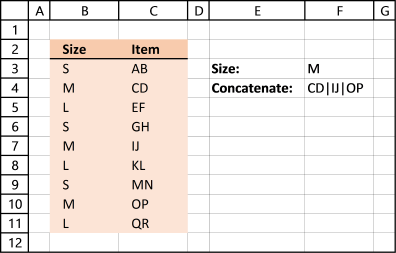Author: Oscar Cronquist Article last updated on February 03, 2023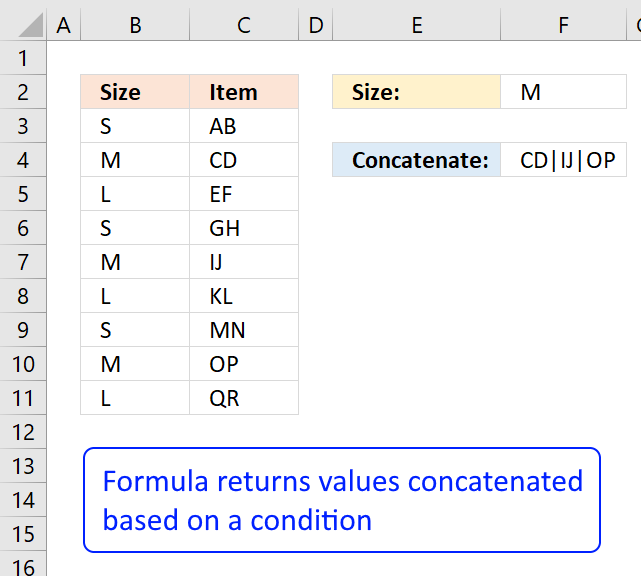The image above shows you data in column B and C. I want to concatenate values on the same row as the condition specified in cell F2 which is in this case "M".

The corresponding values are "CD", "IJ" and "OP", in the picture above.

I highly recommend using the TEXTJOIN function if you use at least Excel 2019 or a later version.

For earlier versions than Excel 2019 use the user-defined function demonstrated here: Lookup and return multiple values concatenated into one cell

It is what the CONCATENATE function should have been from the beginning.

## 1. Create an array of values manually

1. Copy (CTRL + c) this formula:
=TRANSPOSE(IF(B3:B11=F3,C3:C11,""))
2. Double press with left mouse button on cell F4
3. Paste (Ctrl + v) formula to cell F4
4. Select the entire formula in the formula bar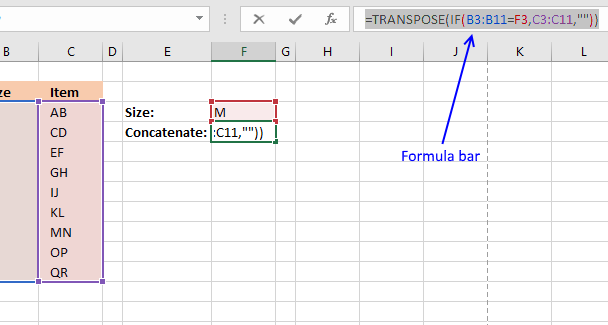5. Press Function key F9 and the formula is converted to an array of constants:
={"","CD","","","IJ","","","OP",""}
6. Delete the equal sign = in the formula bar and then press Enter
{"","CD","","","IJ","","","OP",""}
7. Select cell F4

### 1.1 Explaining formula=TRANSPOSE(IF(B3:B11=F3,C3:C11,""))

#### Step 1 - Logical expression

The equal sign is a logical operator that allows you to compare value to value. In this case, we are going to compare a single value to multiple values.

B3:B11=F3

becomes

{"S";"M";"L";"S";"M";"L";"S";"M";"L"}="M"

The result is TRUE or FALSE based on if the value is equal or not. TRUE and FALSE are boolean values that can be used in a IF function.

{"S";"M";"L";"S";"M";"L";"S";"M";"L"}="M"

returns

{FALSE; TRUE; FALSE; FALSE; TRUE; FALSE; FALSE; TRUE; FALSE}.

#### Step 2 - IF function

The IF function returns one value if the logical test is TRUE and another value if the logical test is FALSE.

IF(B3:B11=F3,C3:C11,"")

becomes

IF({FALSE; TRUE; FALSE; FALSE; TRUE; FALSE; FALSE; TRUE; FALSE}, {"AB";"CD";"EF";"GH";"IJ";"KL";"MN";"OP";"QR"},"")

and returns {"";"CD";"";"";"IJ";"";"";"OP";""}.

#### Step 3 - TRANSPOSE function

The TRANSPOSE function allows you to convert a vertical range to a horizontal range, or vice versa. The CONCATENATE function needs the comma as a delimiting character to function properly.

TRANSPOSE(IF(B3:B11=F3,C3:C11,""))

becomes

TRANSPOSE({"";"CD";"";"";"IJ";"";"";"OP";""})

and returns

{"", "CD", "", "", "IJ", "", "", "OP", ""}.

## 2. Delete blanks in the array

1. I am now going to delete all empty characters in the array, press CTRL + H to open "Search and Replace" Dialog box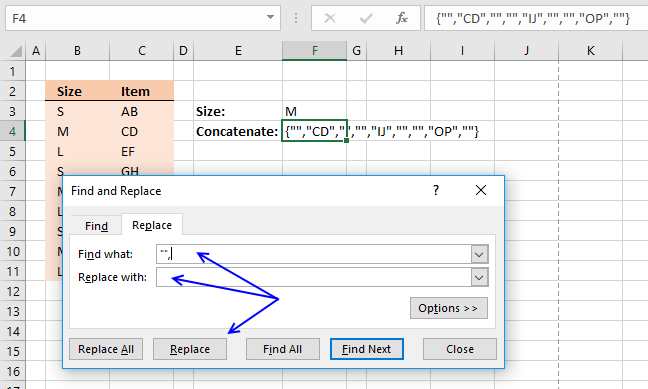2. Type in Find what: "", and nothing in Replace with:
3. Press with mouse on "Replace" button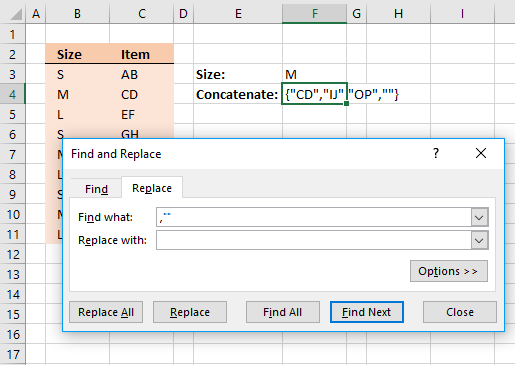4. Type in Find what: ,"" and nothing in Replace with:
5. Press with left mouse button on "Replace" button once again.
6. Press with left mouse button on Close button.

## 3. Concatenate all values in the array

The final thing to do is to use the concatenate function to add all values into one text string.

1. Double press with left mouse button on cell F4
2. Delete the curly brackets from the formula: {}
4. and then an ending parentheses )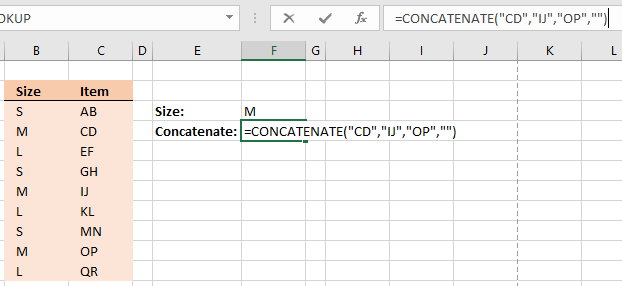5. Press Enter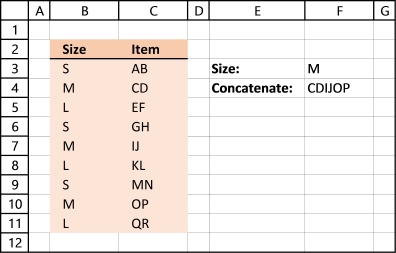Perhaps you want a delimiting character between values, right after you have converted the formula to an array of constants (step 5, above), do this:

1. The array looks like this: ={"","CD","","","IJ","","","OP",""}
2. Add an ampersand and then a delimiting character with quotation marks.
={"","CD","","","IJ","","","OP",""}&"|"
3. Select the formula and press function key F9, the formula now looks like this:
={"|","CD|","|","|","IJ|","|","|","OP|","|"}
The ampersand has added the delimiting character to all values in the array.
4. Continue with step 6 above. Tip! To delete empty values in array, Search and Replace with these values "|", and ,"|"